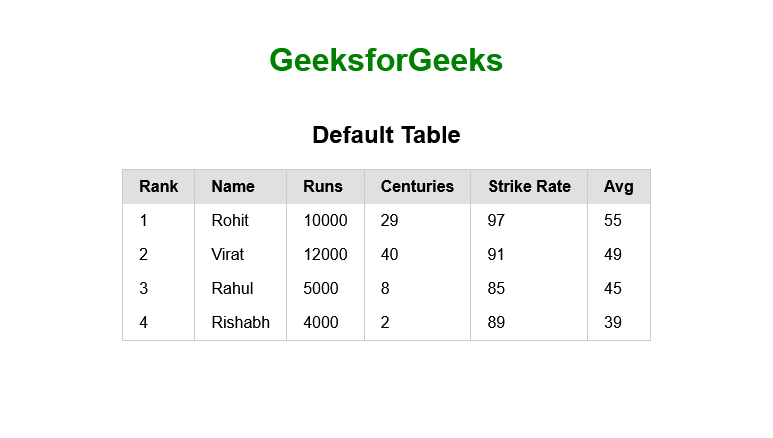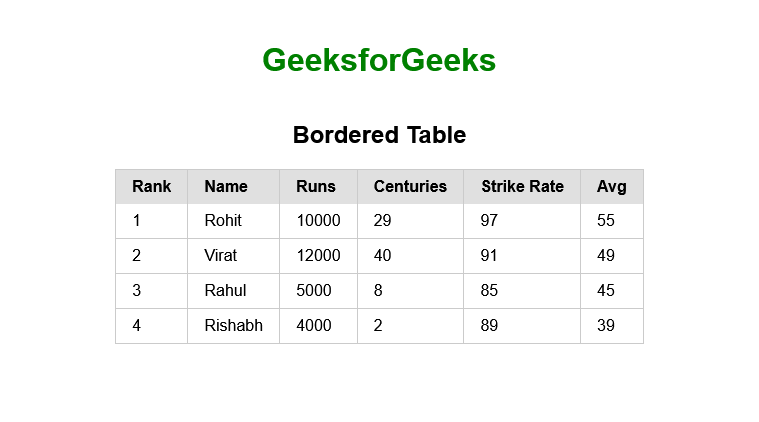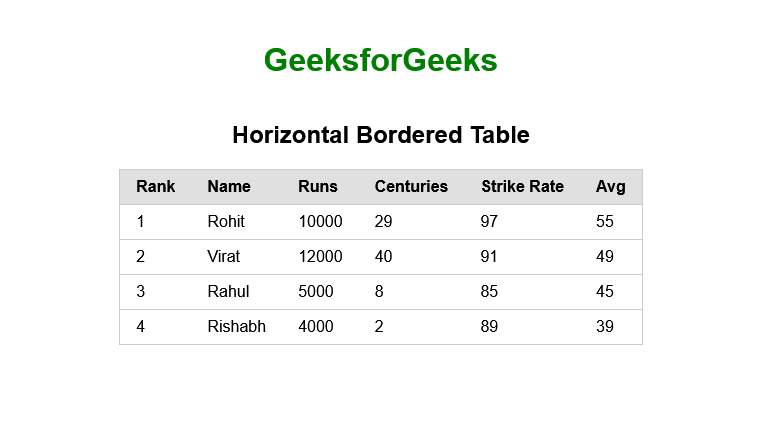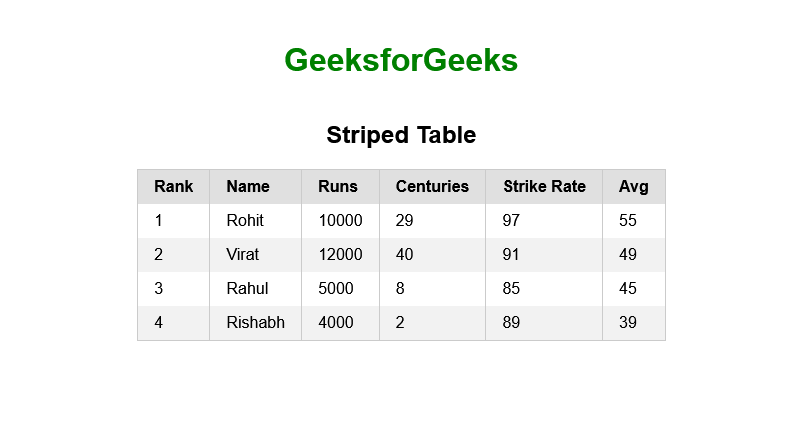Open in App
Not now

# Pure CSS Tables

• Difficulty Level : Hard
• Last Updated : 28 Sep, 2021

Introduction: Before starting with Pure we must know the basics of plain CSS. Basically, Pure CSS is a Cascading Style Sheet framework developed by YAHOO. The main reason for developing Pure CSS is used to develop responsive and reactive websites like Bootstrap which is also compatible with mobile devices and it is an open-source CSS framework and free to use. It can be the best alternative to Materialize CSS.

In this article, we will understand what is Pure CSS Table is? and learn how to use it in our project.

As we think of creating web pages, we know that tables are a nice and easy way to organize a lot of data in websites into the form of rows and columns. By using the Pure CSS framework, we will be able to design different types of tables.

Pure CSS provides a number of utility classes to style tables, there basically 5 main classes as listed below:

• pure-table: This class is used to style the table with default padding and border assign to table elements with an emphasized header.
• pure-table-bordered: This class is used to draw borders on the table vertical and horizontal to all the table cells.
• pure-table-horizontal: This class is used to draw the table with only horizontal lines.
• pure-table-odd: This class is used to create a striped table with the zebra-styled effect which is more visible and attractive for the users.
• pure-table-striped: Basically it is used to display striped tables. It Automatically Striped a table after pass to the <table> element alongside the pure-table class.

Examples: Now here we learn how to use table classes in HTML.

1. Default Table: The pure-table class is used in <table> tag to create a basic table with some basic CSS styling, these tables are just like the normal HTML tables with some padding and border added to table elements with the header.

## HTML

 ```<``html``>``  ``<``head``>``    ````    ``<``link` `rel``=``"stylesheet"``          ``href``=``"https://unpkg.com/purecss@2.0.6/build/pure-min.css"``          ``integrity``=``"sha384-Uu6IeWbM+gzNVXJcM9XV3SohHtmWE+3VGi496jvgX1jyvDTXfdK+rfZc8C1Aehk5"``          ``crossorigin``=``"anonymous"``          ``origin``=``"anonymous"``    ``/>` `    ````    ``<``meta` `name``=``"viewport"` `content``=``"width=device-width, initial-scale=1.0"` `/>` `    ``<``style``>``      ``.container {``        ``margin-top: 20px;``        ``display: flex;``        ``align-items: center;``        ``justify-content: center;``        ``flex-direction: column;``      ``}``      ``h1 {``        ``color: green;``      ``}``    ````  ````  ``<``body``>``    ``<``div` `class``=``"container"``>``      ``<``h1``>GeeksforGeeks``      ``<``h2``>Default Table``      ` `      ````      ``<``table` `class``=``"pure-table"``>``        ``<``thead``>``          ``<``tr``>``            ``<``th``>Rank``            ``<``th``>Name``            ``<``th``>Runs``            ``<``th``>Centuries``            ``<``th``>Strike Rate``            ``<``th``>Avg``          ````        ``` `        ``<``tbody``>``          ``<``tr``>``            ``<``td``>1``            ``<``td``>Rohit``            ``<``td``>10000``            ``<``td``>29``            ``<``td``>97``            ``<``td``>55``          ``` `          ``<``tr``>``            ``<``td``>2``            ``<``td``>Virat``            ``<``td``>12000``            ``<``td``>40``            ``<``td``>91``            ``<``td``>49``          ``` `          ``<``tr``>``            ``<``td``>3``            ``<``td``>Rahul``            ``<``td``>5000``            ``<``td``>8``            ``<``td``>85``            ``<``td``>45``          ``` `          ``<``tr``>``            ``<``td``>4``            ``<``td``>Rishabh``            ``<``td``>4000``            ``<``td``>2``            ``<``td``>89``            ``<``td``>39``          ````        ````      ````    ````  `````

Output:2. Bordered Table: Here we use the pure-table-bordered class is used to create a bordered table. This class will add vertical and horizontal borders to all the cells of the table.

## HTML

 ```<``html``>``  ``<``head``>``    ````    ``<``link` `rel``=``"stylesheet"``          ``href``=``"https://unpkg.com/purecss@2.0.6/build/pure-min.css"``          ``integrity``=``"sha384-Uu6IeWbM+gzNVXJcM9XV3SohHtmWE+3VGi496jvgX1jyvDTXfdK+rfZc8C1Aehk5"``          ``crossorigin``=``"anonymous"``          ``origin``=``"anonymous"``    ``/>` `    ````    ``<``meta` `name``=``"viewport"` `content``=``"width=device-width, initial-scale=1.0"` `/>` `    ``<``style``>``      ``.container {``        ``margin-top: 20px;``        ``display: flex;``        ``align-items: center;` `        ``justify-content: center;``        ``flex-direction: column;``      ``}``      ``h1 {``        ``color: green;``      ``}``    ````  ````  ``<``body``>``    ``<``div` `class``=``"container"``>``      ``<``h1``>GeeksforGeeks``      ``<``h2``>Bordered Table``      ``` `      ``<``table` `class``=``"pure-table pure-table-bordered"``>``        ``<``thead``>``          ``<``tr``>``            ``<``th``>Rank``            ``<``th``>Name``            ``<``th``>Runs``            ``<``th``>Centuries``            ``<``th``>Strike Rate``            ``<``th``>Avg``          ````        ``` `        ``<``tbody``>``          ``<``tr``>``            ``<``td``>1``            ``<``td``>Rohit``            ``<``td``>10000``            ``<``td``>29``            ``<``td``>97``            ``<``td``>55``          ``` `          ``<``tr``>``            ``<``td``>2``            ``<``td``>Virat``            ``<``td``>12000``            ``<``td``>40``            ``<``td``>91``            ``<``td``>49``          ``` `          ``<``tr``>``            ``<``td``>3``            ``<``td``>Rahul``            ``<``td``>5000``            ``<``td``>8``            ``<``td``>85``            ``<``td``>45``          ``` `          ``<``tr``>``            ``<``td``>4``            ``<``td``>Rishabh``            ``<``td``>4000``            ``<``td``>2``            ``<``td``>89``            ``<``td``>39``          ````        ````      ````    ````  `````

Output:3. Table with Horizontal Borders: Here we used the pure-table-horizontal class to create this type of table. This will create a table with horizontal lines only.

## HTML

 ```<``html``>``  ``<``head``>``    ````    ``<``link` `rel``=``"stylesheet"``          ``href``=``"https://unpkg.com/purecss@2.0.6/build/pure-min.css"``          ``integrity``=``"sha384-Uu6IeWbM+gzNVXJcM9XV3SohHtmWE+3VGi496jvgX1jyvDTXfdK+rfZc8C1Aehk5"``          ``crossorigin``=``"anonymous"``          ``origin``=``"anonymous"``    ``/>` `    ````    ``<``meta` `name``=``"viewport"` `content``=``"width=device-width, initial-scale=1.0"` `/>` `    ``<``style``>``      ``.container {``        ``margin-top: 20px;``        ``display: flex;``        ``align-items: center;` `        ``justify-content: center;``        ``flex-direction: column;``      ``}``      ``h1 {``        ``color: green;``      ``}``    ````  ````  ``<``body``>``    ``<``div` `class``=``"container"``>``      ``<``h1``>GeeksforGeeks``      ``<``h2``>Horizontal Bordered Table` `      ````      ``<``table` `class``=``"pure-table pure-table-horizontal"``>``        ``<``thead``>``          ``<``tr``>``            ``<``th``>Rank``            ``<``th``>Name``            ``<``th``>Runs``            ``<``th``>Centuries``            ``<``th``>Strike Rate``            ``<``th``>Avg``          ````        ``` `        ``<``tbody``>``          ``<``tr``>``            ``<``td``>1``            ``<``td``>Rohit``            ``<``td``>10000``            ``<``td``>29``            ``<``td``>97``            ``<``td``>55``          ``` `          ``<``tr``>``            ``<``td``>2``            ``<``td``>Virat``            ``<``td``>12000``            ``<``td``>40``            ``<``td``>91``            ``<``td``>49``          ``` `          ``<``tr``>``            ``<``td``>3``            ``<``td``>Rahul``            ``<``td``>5000``            ``<``td``>8``            ``<``td``>85``            ``<``td``>45``          ``` `          ``<``tr``>``            ``<``td``>4``            ``<``td``>Rishabh``            ``<``td``>4000``            ``<``td``>2``            ``<``td``>89``            ``<``td``>39``          ````        ````      ````    ````  `````

Output:4. Striped Table: For better visualization of large-size tables we can use striped cells. For a striped table here we used the pure-table-odd Pure CSS class in the every <tr> element tag to change the background of the odd rows. This will create a striped table with the zebra-styled effect which is more visible and attractive for a large number of data to the user.

## HTML

 ```<``html``>``  ``<``head``>``    ````    ``<``link` `rel``=``"stylesheet"``          ``href``=``"https://unpkg.com/purecss@2.0.6/build/pure-min.css"``          ``integrity``=``"sha384-Uu6IeWbM+gzNVXJcM9XV3SohHtmWE+3VGi496jvgX1jyvDTXfdK+rfZc8C1Aehk5"``          ``crossorigin``=``"anonymous"``          ``origin``=``"anonymous"``    ``/>` `    ````    ``<``meta` `name``=``"viewport"` `content``=``"width=device-width, initial-scale=1.0"` `/>` `    ``<``style``>``      ``.container {``        ``margin-top: 20px;``        ``display: flex;``        ``align-items: center;``        ``justify-content: center;``        ``flex-direction: column;``      ``}``      ``h1 {``        ``color: green;``      ``}``    ````  ````  ``<``body``>``    ``<``div` `class``=``"container"``>``      ``<``h1``>GeeksforGeeks``      ``<``h2``>Horizontal Bordered Table` `      ````      ``<``table` `class``=``"pure-table"``>``        ``<``thead``>``          ``<``tr``>``            ``<``th``>Rank``            ``<``th``>Name``            ``<``th``>Runs``            ``<``th``>Centuries``            ``<``th``>Strike Rate``            ``<``th``>Avg``          ````        ``` `        ``<``tbody``>``          ``<``tr``>``            ``<``td``>1``            ``<``td``>Rohit``            ``<``td``>10000``            ``<``td``>29``            ``<``td``>97``            ``<``td``>55``          ``` `          ````          ``<``tr` `class``=``"pure-table-odd"``>``            ``<``td``>2``            ``<``td``>Virat``            ``<``td``>12000``            ``<``td``>40``            ``<``td``>91``            ``<``td``>49``          ``` `          ``<``tr``>``            ``<``td``>3``            ``<``td``>Rahul``            ``<``td``>5000``            ``<``td``>8``            ``<``td``>85``            ``<``td``>45``          ``` `          ``<``tr` `class``=``"pure-table-odd"``>``            ``<``td``>4``            ``<``td``>Rishabh``            ``<``td``>4000``            ``<``td``>2``            ``<``td``>89``            ``<``td``>39``          ````        ````      ````    ````  `````

Output:In this article, we learn what is Pure CSS Table is, its classes, and how to use it in various tables.

My Personal Notes arrow_drop_up